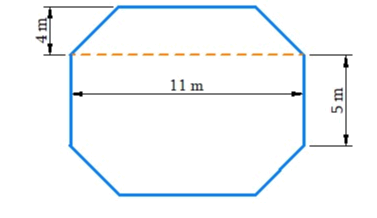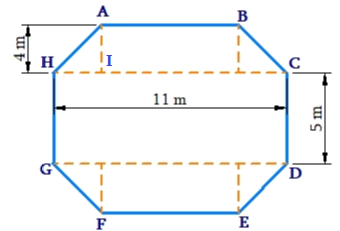# Ex.11.2 Q9 Mensuration Solution - NCERT Maths Class 8

## Question

Top surface of a raised platform is in the shape of a regular octagon as shown in the figure. Find the area of the octagonal surface.Video Solution
Mensuration
Ex 11.2 | Question 9

## Text Solution

What is Known?

Top surface of a raised platform is octagonal shaped.

What is unknown?

Area of the octagonal surfaceReasoning:

Visually, the area of the octagonal surface will be the sum of the area of two trapezium and area of rectangular.

Steps:

Area of octagon $$ABCDEFGH =$$ Area of trapezium $$ABCH\, +$$ Area of rectangular $$HCDG \;+$$ Area of trapezium $$EFGD$$

Side of the regular octagon $$= 5\,\rm{cm}$$

Area of trapezium $$ABCH\, =\,$$ Area of trapezium $$EFGD$$

Area of trapezium $$ABCH$$

\begin{align} &= \frac{1}{2} \times (AB + CH) \times AI\\&= \frac{1}{2} \times (5\,\rm{m} + 11\,\rm{m}) \times 4\,\rm{m}\\&= \frac{1}{2} \times 16\,\rm{m} \times 4\,\rm{m}\\&= 32\,\rm{m^2}\end{align}

$$\therefore$$ Area of trapezium $$ABCH\,=\,$$ Area of trapezium $$EFGD= 32 \rm{m^2}$$

Area of rectangle $$HCDG$$

\begin{align} &= HC \times CD \\&= 11{\rm{m}} \times 5{\rm{m}}\\& = 55{{\rm{m}}^2}\end{align}

Area of $$ABCDEFGH$$ $$=$$ Area of trapezium $$ABCH$$ $$+$$ Area of rectangle $$HCDG$$ $$+$$ Area of trapezium $$EFGD$$

\begin{align}& = 32\,{{\rm{m}}^2} + 55\,{{\rm{m}}^2} + 32\,{{\rm{m}}^2}\\& = 119\,\rm{m^2}\end{align}

Thus, the area of the octagonal surface is $$119{{\rm{m}}^2}$$

Learn from the best math teachers and top your exams

• Live one on one classroom and doubt clearing
• Practice worksheets in and after class for conceptual clarity
• Personalized curriculum to keep up with school